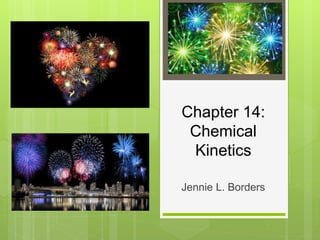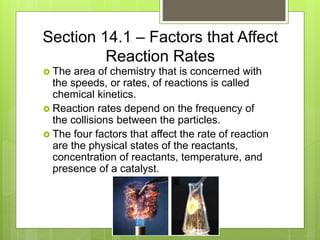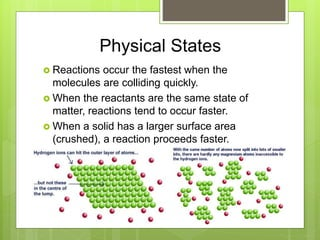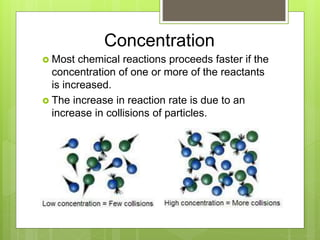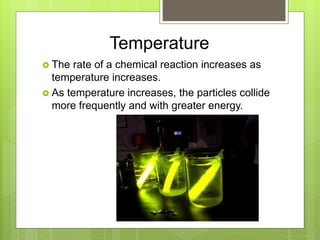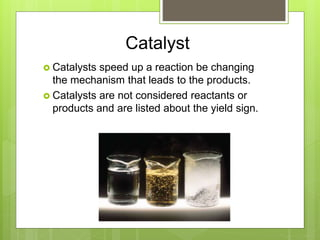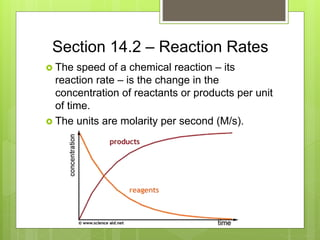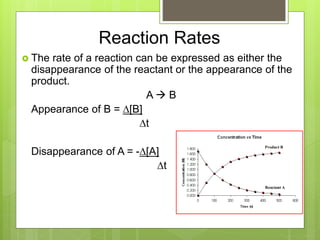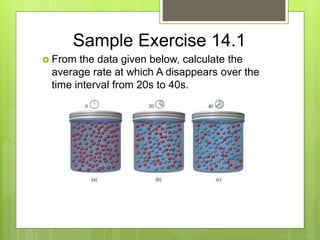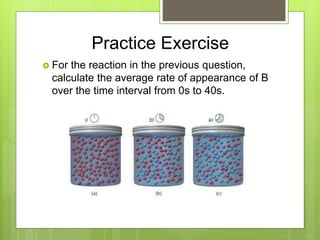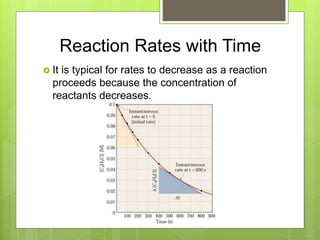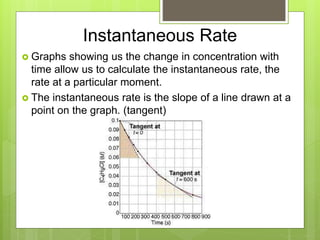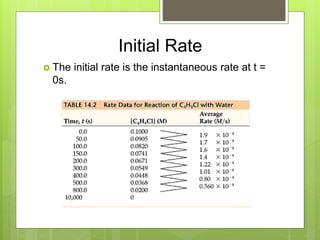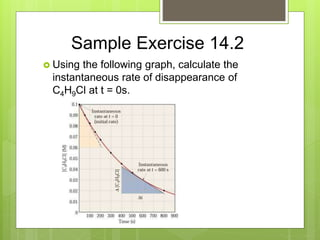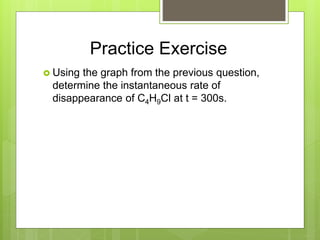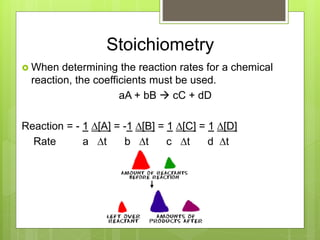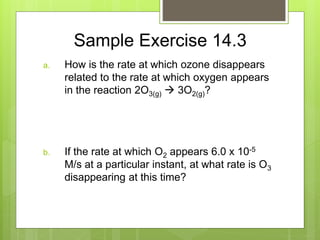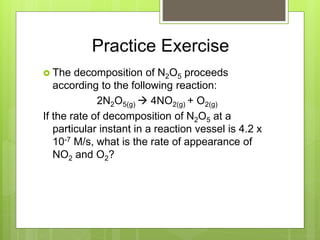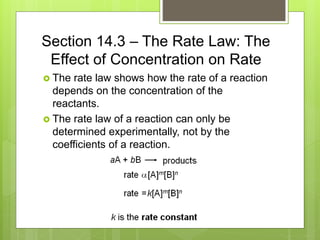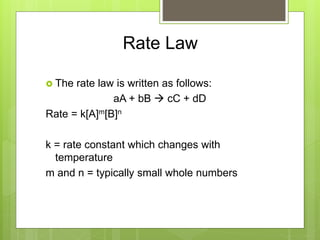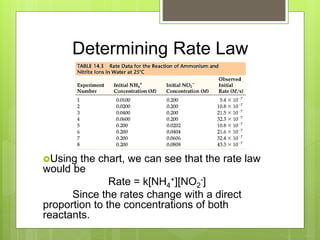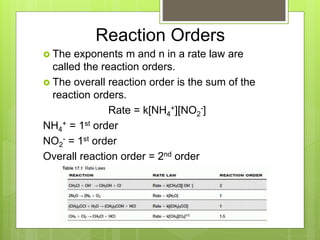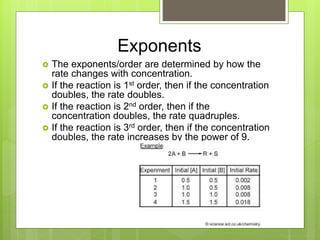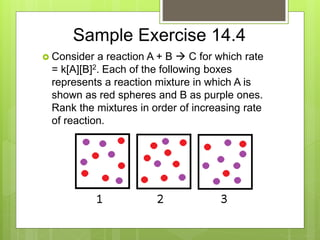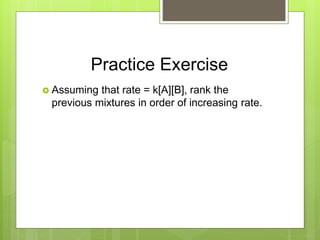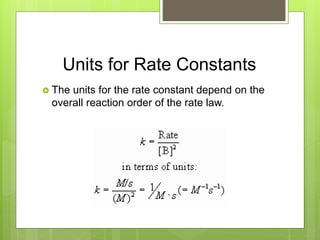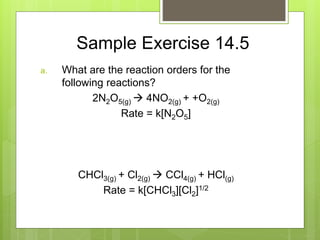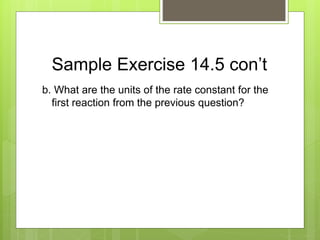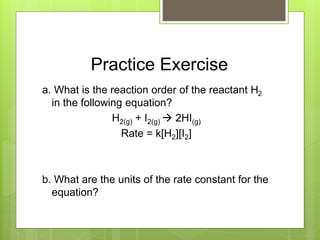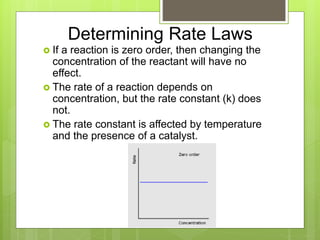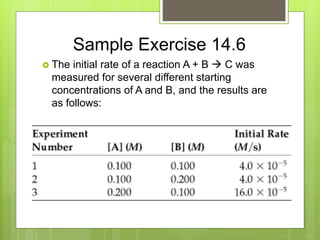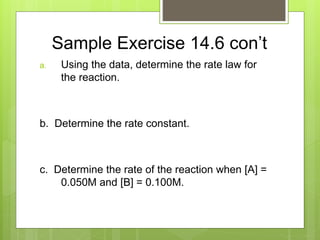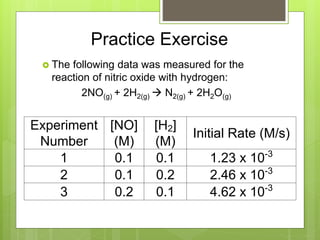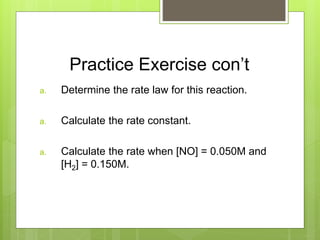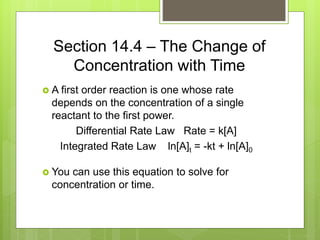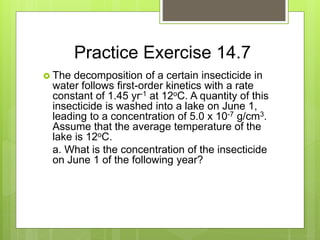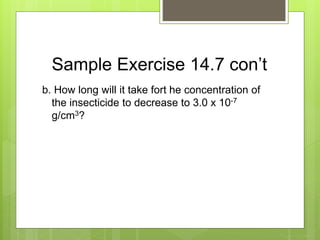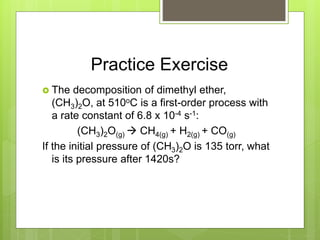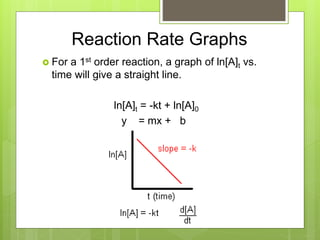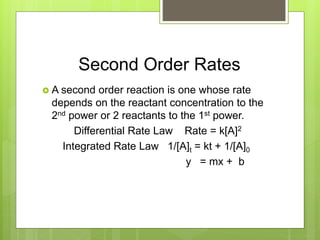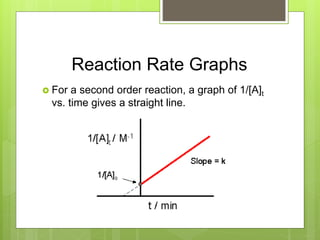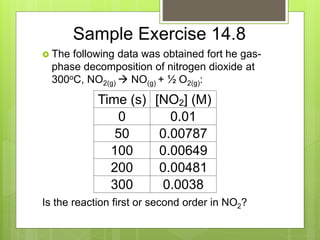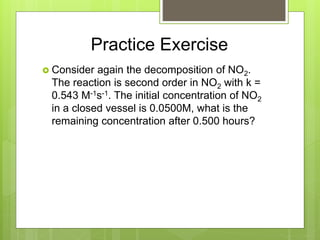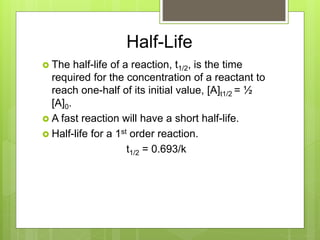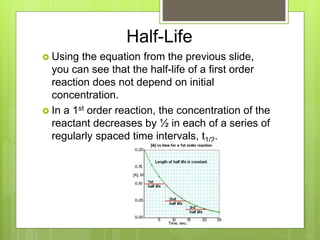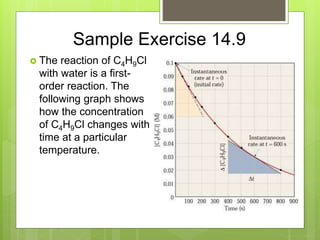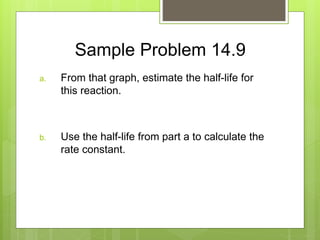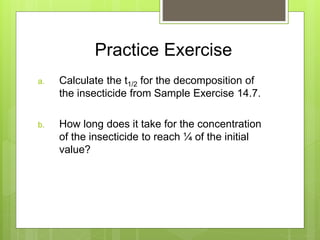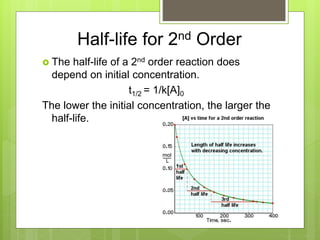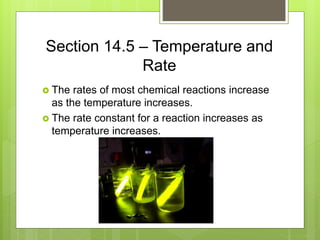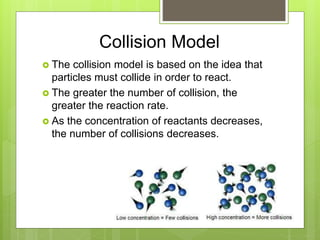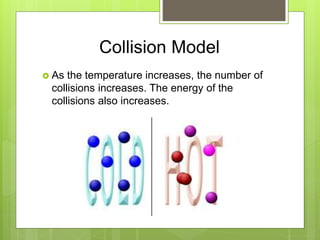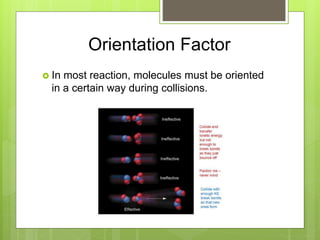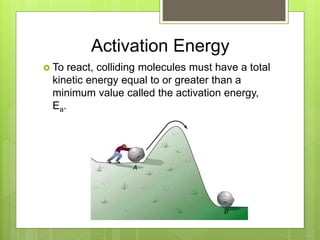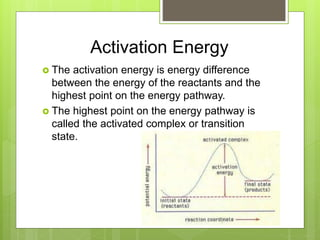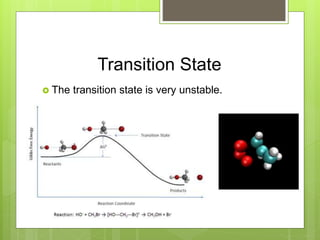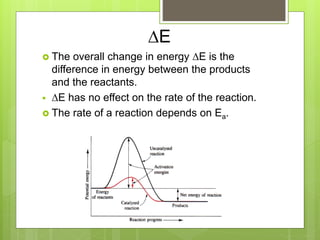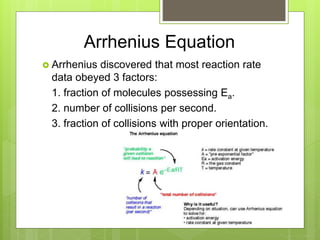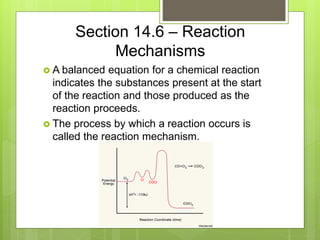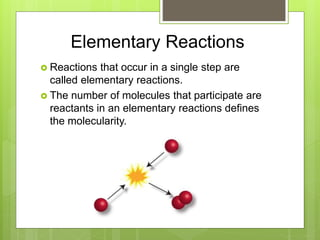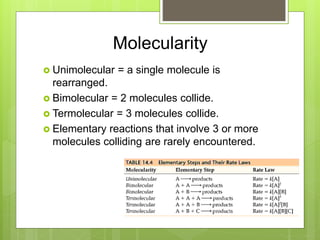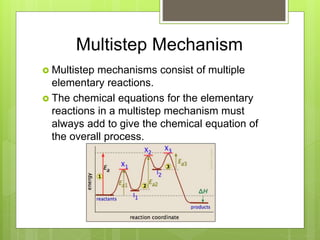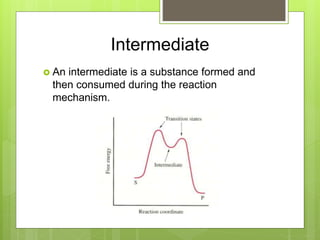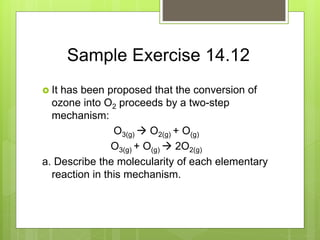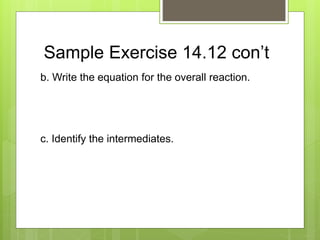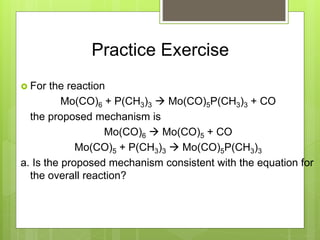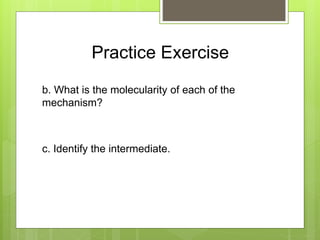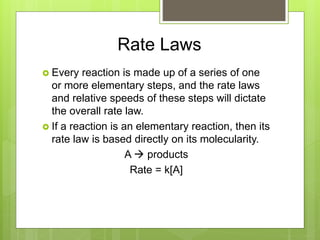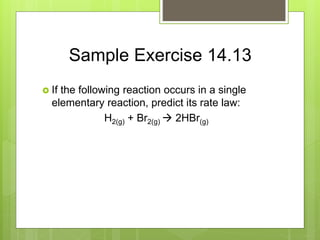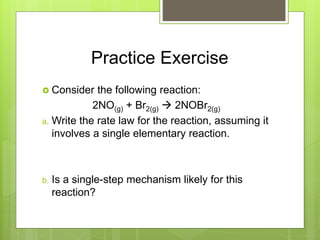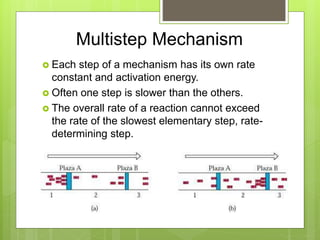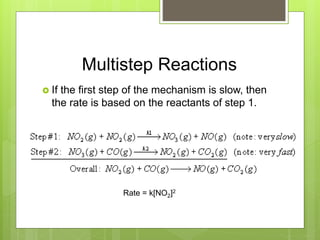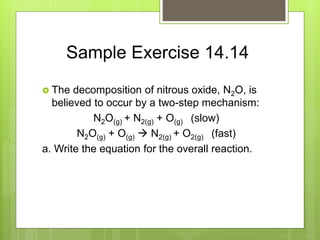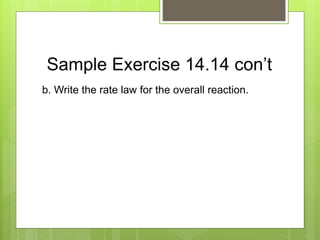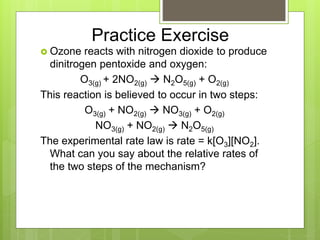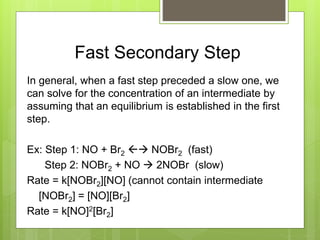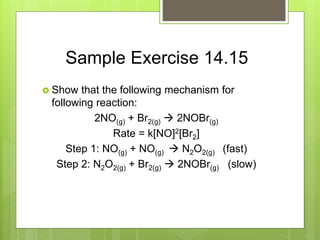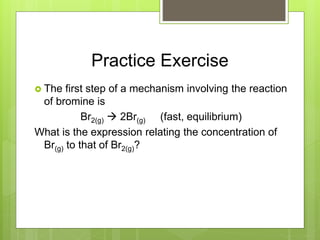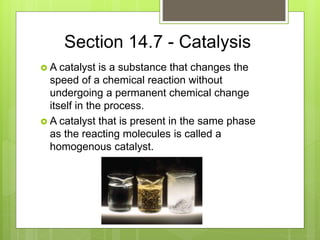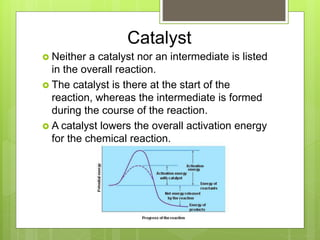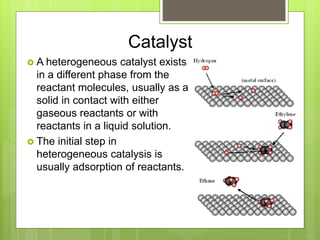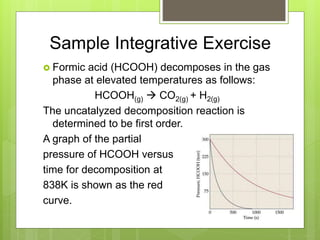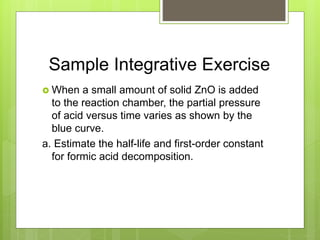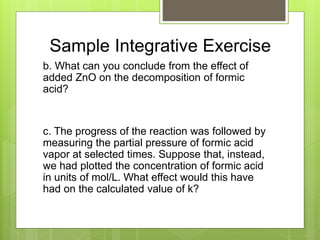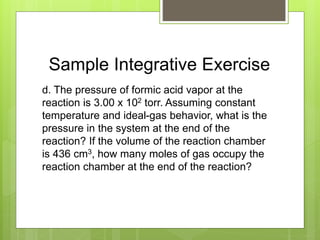1 of 86

### AP Chemistry Chapter 14 Powerpoint for website.ppt

1. Chapter 14: Chemical Kinetics Jennie L. Borders
2. Section 14.1 – Factors that Affect Reaction Rates  The area of chemistry that is concerned with the speeds, or rates, of reactions is called chemical kinetics.  Reaction rates depend on the frequency of the collisions between the particles.  The four factors that affect the rate of reaction are the physical states of the reactants, concentration of reactants, temperature, and presence of a catalyst.
3. Physical States  Reactions occur the fastest when the molecules are colliding quickly.  When the reactants are the same state of matter, reactions tend to occur faster.  When a solid has a larger surface area (crushed), a reaction proceeds faster.
4. Concentration  Most chemical reactions proceeds faster if the concentration of one or more of the reactants is increased.  The increase in reaction rate is due to an increase in collisions of particles.
5. Temperature  The rate of a chemical reaction increases as temperature increases.  As temperature increases, the particles collide more frequently and with greater energy.
6. Catalyst  Catalysts speed up a reaction be changing the mechanism that leads to the products.  Catalysts are not considered reactants or products and are listed about the yield sign.
7. Section 14.2 – Reaction Rates  The speed of a chemical reaction – its reaction rate – is the change in the concentration of reactants or products per unit of time.  The units are molarity per second (M/s).
8. Reaction Rates  The rate of a reaction can be expressed as either the disappearance of the reactant or the appearance of the product. A  B Appearance of B = D[B] Dt Disappearance of A = -D[A] Dt
9. Sample Exercise 14.1  From the data given below, calculate the average rate at which A disappears over the time interval from 20s to 40s.
10. Practice Exercise  For the reaction in the previous question, calculate the average rate of appearance of B over the time interval from 0s to 40s.
11. Reaction Rates with Time  It is typical for rates to decrease as a reaction proceeds because the concentration of reactants decreases.
12. Instantaneous Rate  Graphs showing us the change in concentration with time allow us to calculate the instantaneous rate, the rate at a particular moment.  The instantaneous rate is the slope of a line drawn at a point on the graph. (tangent)
13. Initial Rate  The initial rate is the instantaneous rate at t = 0s.
14. Sample Exercise 14.2  Using the following graph, calculate the instantaneous rate of disappearance of C4H9Cl at t = 0s.
15. Practice Exercise  Using the graph from the previous question, determine the instantaneous rate of disappearance of C4H9Cl at t = 300s.
16. Stoichiometry  When determining the reaction rates for a chemical reaction, the coefficients must be used. aA + bB  cC + dD Reaction = - 1 D[A] = -1 D[B] = 1 D[C] = 1 D[D] Rate a Dt b Dt c Dt d Dt
17. Sample Exercise 14.3 a. How is the rate at which ozone disappears related to the rate at which oxygen appears in the reaction 2O3(g)  3O2(g)? b. If the rate at which O2 appears 6.0 x 10-5 M/s at a particular instant, at what rate is O3 disappearing at this time?
18. Practice Exercise  The decomposition of N2O5 proceeds according to the following reaction: 2N2O5(g)  4NO2(g) + O2(g) If the rate of decomposition of N2O5 at a particular instant in a reaction vessel is 4.2 x 10-7 M/s, what is the rate of appearance of NO2 and O2?
19. Section 14.3 – The Rate Law: The Effect of Concentration on Rate  The rate law shows how the rate of a reaction depends on the concentration of the reactants.  The rate law of a reaction can only be determined experimentally, not by the coefficients of a reaction.
20. Rate Law  The rate law is written as follows: aA + bB  cC + dD Rate = k[A]m[B]n k = rate constant which changes with temperature m and n = typically small whole numbers
21. Determining Rate Law Using the chart, we can see that the rate law would be Rate = k[NH4 +][NO2 -] Since the rates change with a direct proportion to the concentrations of both reactants.
22. Reaction Orders  The exponents m and n in a rate law are called the reaction orders.  The overall reaction order is the sum of the reaction orders. Rate = k[NH4 +][NO2 -] NH4 + = 1st order NO2 - = 1st order Overall reaction order = 2nd order
23. Exponents  The exponents/order are determined by how the rate changes with concentration.  If the reaction is 1st order, then if the concentration doubles, the rate doubles.  If the reaction is 2nd order, then if the concentration doubles, the rate quadruples.  If the reaction is 3rd order, then if the concentration doubles, the rate increases by the power of 9.
24. Sample Exercise 14.4  Consider a reaction A + B  C for which rate = k[A][B]2. Each of the following boxes represents a reaction mixture in which A is shown as red spheres and B as purple ones. Rank the mixtures in order of increasing rate of reaction.
25. Practice Exercise  Assuming that rate = k[A][B], rank the previous mixtures in order of increasing rate.
26. Units for Rate Constants  The units for the rate constant depend on the overall reaction order of the rate law.
27. Sample Exercise 14.5 a. What are the reaction orders for the following reactions? 2N2O5(g)  4NO2(g) + +O2(g) Rate = k[N2O5] CHCl3(g) + Cl2(g)  CCl4(g) + HCl(g) Rate = k[CHCl3][Cl2]1/2
28. Sample Exercise 14.5 con’t b. What are the units of the rate constant for the first reaction from the previous question?
29. Practice Exercise a. What is the reaction order of the reactant H2 in the following equation? H2(g) + I2(g)  2HI(g) Rate = k[H2][I2] b. What are the units of the rate constant for the equation?
30. Determining Rate Laws  If a reaction is zero order, then changing the concentration of the reactant will have no effect.  The rate of a reaction depends on concentration, but the rate constant (k) does not.  The rate constant is affected by temperature and the presence of a catalyst.
31. Sample Exercise 14.6  The initial rate of a reaction A + B  C was measured for several different starting concentrations of A and B, and the results are as follows:
32. Sample Exercise 14.6 con’t a. Using the data, determine the rate law for the reaction. b. Determine the rate constant. c. Determine the rate of the reaction when [A] = 0.050M and [B] = 0.100M.
33. Practice Exercise  The following data was measured for the reaction of nitric oxide with hydrogen: 2NO(g) + 2H2(g)  N2(g) + 2H2O(g) Experiment Number [NO] (M) [H2] (M) Initial Rate (M/s) 1 0.1 0.1 1.23 x 10-3 2 0.1 0.2 2.46 x 10-3 3 0.2 0.1 4.62 x 10-3
34. Practice Exercise con’t a. Determine the rate law for this reaction. a. Calculate the rate constant. a. Calculate the rate when [NO] = 0.050M and [H2] = 0.150M.
35. Section 14.4 – The Change of Concentration with Time  A first order reaction is one whose rate depends on the concentration of a single reactant to the first power. Differential Rate Law Rate = k[A] Integrated Rate Law ln[A]t = -kt + ln[A]0  You can use this equation to solve for concentration or time.
36. Practice Exercise 14.7  The decomposition of a certain insecticide in water follows first-order kinetics with a rate constant of 1.45 yr-1 at 12oC. A quantity of this insecticide is washed into a lake on June 1, leading to a concentration of 5.0 x 10-7 g/cm3. Assume that the average temperature of the lake is 12oC. a. What is the concentration of the insecticide on June 1 of the following year?
37. Sample Exercise 14.7 con’t b. How long will it take fort he concentration of the insecticide to decrease to 3.0 x 10-7 g/cm3?
38. Practice Exercise  The decomposition of dimethyl ether, (CH3)2O, at 510oC is a first-order process with a rate constant of 6.8 x 10-4 s-1: (CH3)2O(g)  CH4(g) + H2(g) + CO(g) If the initial pressure of (CH3)2O is 135 torr, what is its pressure after 1420s?
39. Reaction Rate Graphs  For a 1st order reaction, a graph of ln[A]t vs. time will give a straight line. ln[A]t = -kt + ln[A]0 y = mx + b
40. Second Order Rates  A second order reaction is one whose rate depends on the reactant concentration to the 2nd power or 2 reactants to the 1st power. Differential Rate Law Rate = k[A]2 Integrated Rate Law 1/[A]t = kt + 1/[A]0 y = mx + b
41. Reaction Rate Graphs  For a second order reaction, a graph of 1/[A]t vs. time gives a straight line.
42. Sample Exercise 14.8  The following data was obtained fort he gas- phase decomposition of nitrogen dioxide at 300oC, NO2(g)  NO(g) + ½ O2(g): Is the reaction first or second order in NO2? Time (s) [NO2] (M) 0 0.01 50 0.00787 100 0.00649 200 0.00481 300 0.0038
43. Practice Exercise  Consider again the decomposition of NO2. The reaction is second order in NO2 with k = 0.543 M-1s-1. The initial concentration of NO2 in a closed vessel is 0.0500M, what is the remaining concentration after 0.500 hours?
44. Half-Life  The half-life of a reaction, t1/2, is the time required for the concentration of a reactant to reach one-half of its initial value, [A]t1/2 = ½ [A]0.  A fast reaction will have a short half-life.  Half-life for a 1st order reaction. t1/2 = 0.693/k
45. Half-Life  Using the equation from the previous slide, you can see that the half-life of a first order reaction does not depend on initial concentration.  In a 1st order reaction, the concentration of the reactant decreases by ½ in each of a series of regularly spaced time intervals, t1/2.
46. Sample Exercise 14.9  The reaction of C4H9Cl with water is a first- order reaction. The following graph shows how the concentration of C4H9Cl changes with time at a particular temperature.
47. Sample Problem 14.9 a. From that graph, estimate the half-life for this reaction. b. Use the half-life from part a to calculate the rate constant.
48. Practice Exercise a. Calculate the t1/2 for the decomposition of the insecticide from Sample Exercise 14.7. b. How long does it take for the concentration of the insecticide to reach ¼ of the initial value?
49. Half-life for 2nd Order  The half-life of a 2nd order reaction does depend on initial concentration. t1/2 = 1/k[A]0 The lower the initial concentration, the larger the half-life.
50. Section 14.5 – Temperature and Rate  The rates of most chemical reactions increase as the temperature increases.  The rate constant for a reaction increases as temperature increases.
51. Collision Model  The collision model is based on the idea that particles must collide in order to react.  The greater the number of collision, the greater the reaction rate.  As the concentration of reactants decreases, the number of collisions decreases.
52. Collision Model  As the temperature increases, the number of collisions increases. The energy of the collisions also increases.
53. Orientation Factor  In most reaction, molecules must be oriented in a certain way during collisions.
54. Activation Energy  To react, colliding molecules must have a total kinetic energy equal to or greater than a minimum value called the activation energy, Ea.
55. Activation Energy  The activation energy is energy difference between the energy of the reactants and the highest point on the energy pathway.  The highest point on the energy pathway is called the activated complex or transition state.
56. Transition State  The transition state is very unstable.
57. DE  The overall change in energy DE is the difference in energy between the products and the reactants.  DE has no effect on the rate of the reaction.  The rate of a reaction depends on Ea.
58. Arrhenius Equation  Arrhenius discovered that most reaction rate data obeyed 3 factors: 1. fraction of molecules possessing Ea. 2. number of collisions per second. 3. fraction of collisions with proper orientation.
59. Section 14.6 – Reaction Mechanisms  A balanced equation for a chemical reaction indicates the substances present at the start of the reaction and those produced as the reaction proceeds.  The process by which a reaction occurs is called the reaction mechanism.
60. Elementary Reactions  Reactions that occur in a single step are called elementary reactions.  The number of molecules that participate are reactants in an elementary reactions defines the molecularity.
61. Molecularity  Unimolecular = a single molecule is rearranged.  Bimolecular = 2 molecules collide.  Termolecular = 3 molecules collide.  Elementary reactions that involve 3 or more molecules colliding are rarely encountered.
62. Multistep Mechanism  Multistep mechanisms consist of multiple elementary reactions.  The chemical equations for the elementary reactions in a multistep mechanism must always add to give the chemical equation of the overall process.
63. Intermediate  An intermediate is a substance formed and then consumed during the reaction mechanism.
64. Sample Exercise 14.12  It has been proposed that the conversion of ozone into O2 proceeds by a two-step mechanism: O3(g)  O2(g) + O(g) O3(g) + O(g)  2O2(g) a. Describe the molecularity of each elementary reaction in this mechanism.
65. Sample Exercise 14.12 con’t b. Write the equation for the overall reaction. c. Identify the intermediates.
66. Practice Exercise  For the reaction Mo(CO)6 + P(CH3)3  Mo(CO)5P(CH3)3 + CO the proposed mechanism is Mo(CO)6  Mo(CO)5 + CO Mo(CO)5 + P(CH3)3  Mo(CO)5P(CH3)3 a. Is the proposed mechanism consistent with the equation for the overall reaction?
67. Practice Exercise b. What is the molecularity of each of the mechanism? c. Identify the intermediate.
68. Rate Laws  Every reaction is made up of a series of one or more elementary steps, and the rate laws and relative speeds of these steps will dictate the overall rate law.  If a reaction is an elementary reaction, then its rate law is based directly on its molecularity. A  products Rate = k[A]
69. Sample Exercise 14.13  If the following reaction occurs in a single elementary reaction, predict its rate law: H2(g) + Br2(g)  2HBr(g)
70. Practice Exercise  Consider the following reaction: 2NO(g) + Br2(g)  2NOBr2(g) a. Write the rate law for the reaction, assuming it involves a single elementary reaction. b. Is a single-step mechanism likely for this reaction?
71. Multistep Mechanism  Each step of a mechanism has its own rate constant and activation energy.  Often one step is slower than the others.  The overall rate of a reaction cannot exceed the rate of the slowest elementary step, rate- determining step.
72. Multistep Reactions  If the first step of the mechanism is slow, then the rate is based on the reactants of step 1. Rate = k[NO2]2
73. Sample Exercise 14.14  The decomposition of nitrous oxide, N2O, is believed to occur by a two-step mechanism: N2O(g) + N2(g) + O(g) (slow) N2O(g) + O(g)  N2(g) + O2(g) (fast) a. Write the equation for the overall reaction.
74. Sample Exercise 14.14 con’t b. Write the rate law for the overall reaction.
75. Practice Exercise  Ozone reacts with nitrogen dioxide to produce dinitrogen pentoxide and oxygen: O3(g) + 2NO2(g)  N2O5(g) + O2(g) This reaction is believed to occur in two steps: O3(g) + NO2(g)  NO3(g) + O2(g) NO3(g) + NO2(g)  N2O5(g) The experimental rate law is rate = k[O3][NO2]. What can you say about the relative rates of the two steps of the mechanism?
76. Fast Secondary Step In general, when a fast step preceded a slow one, we can solve for the concentration of an intermediate by assuming that an equilibrium is established in the first step. Ex: Step 1: NO + Br2  NOBr2 (fast) Step 2: NOBr2 + NO  2NOBr (slow) Rate = k[NOBr2][NO] (cannot contain intermediate [NOBr2] = [NO][Br2] Rate = k[NO]2[Br2]
77. Sample Exercise 14.15  Show that the following mechanism for following reaction: 2NO(g) + Br2(g)  2NOBr(g) Rate = k[NO]2[Br2] Step 1: NO(g) + NO(g)  N2O2(g) (fast) Step 2: N2O2(g) + Br2(g)  2NOBr(g) (slow)
78. Practice Exercise  The first step of a mechanism involving the reaction of bromine is Br2(g)  2Br(g) (fast, equilibrium) What is the expression relating the concentration of Br(g) to that of Br2(g)?
79. Section 14.7 - Catalysis  A catalyst is a substance that changes the speed of a chemical reaction without undergoing a permanent chemical change itself in the process.  A catalyst that is present in the same phase as the reacting molecules is called a homogenous catalyst.
80. Catalyst  Neither a catalyst nor an intermediate is listed in the overall reaction.  The catalyst is there at the start of the reaction, whereas the intermediate is formed during the course of the reaction.  A catalyst lowers the overall activation energy for the chemical reaction.
81. Catalyst  A heterogeneous catalyst exists in a different phase from the reactant molecules, usually as a solid in contact with either gaseous reactants or with reactants in a liquid solution.  The initial step in heterogeneous catalysis is usually adsorption of reactants.
82. Sample Integrative Exercise  Formic acid (HCOOH) decomposes in the gas phase at elevated temperatures as follows: HCOOH(g)  CO2(g) + H2(g) The uncatalyzed decomposition reaction is determined to be first order. A graph of the partial pressure of HCOOH versus time for decomposition at 838K is shown as the red curve.
83. Sample Integrative Exercise  When a small amount of solid ZnO is added to the reaction chamber, the partial pressure of acid versus time varies as shown by the blue curve. a. Estimate the half-life and first-order constant for formic acid decomposition.
84. Sample Integrative Exercise b. What can you conclude from the effect of added ZnO on the decomposition of formic acid? c. The progress of the reaction was followed by measuring the partial pressure of formic acid vapor at selected times. Suppose that, instead, we had plotted the concentration of formic acid in units of mol/L. What effect would this have had on the calculated value of k?
85. Sample Integrative Exercise d. The pressure of formic acid vapor at the reaction is 3.00 x 102 torr. Assuming constant temperature and ideal-gas behavior, what is the pressure in the system at the end of the reaction? If the volume of the reaction chamber is 436 cm3, how many moles of gas occupy the reaction chamber at the end of the reaction?
86. Sample Integrative Exercise  e. The standard heat of formation of formic acid vapor is DHo f = -378.6 kJ/mol. Calculate DHo for the overall reaction. If the activation energy (Ea) for the reaction is 184 kJ/mol, sketch an approximate energy profile for the reaction, and label Ea, DHo, and the transition state.